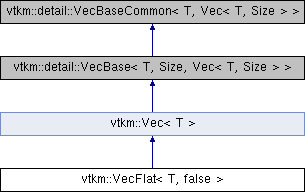VTK-m  1.6
vtkm::VecFlat< T, false > Class Template Reference

#include <VecFlat.h>

Inheritance diagram for vtkm::VecFlat< T, false >:Public Member Functions

VecFlat ()=default

VTKM_EXEC_CONT VecFlat (const T &src)

VTKM_EXEC_CONT VecFlatoperator= (const T &src)

VTKM_EXEC_CONT operator T () constPublic Member Functions inherited from vtkm::Vec< T, Size >
Vec ()=default

VTKM_EXEC_CONT void CopyInto (Vec< T, Size > &dest) const

Private Types

using Superclass = internal::FlattenVec< T >Public Types inherited from vtkm::Vec< T, Size >
using ComponentType = TStatic Public Attributes inherited from vtkm::Vec< T, Size >
static constexpr vtkm::IdComponent NUM_COMPONENTS = Size

◆ Superclass

template<typename T >
 using vtkm::VecFlat< T, false >::Superclass = internal::FlattenVec
private

◆ VecFlat() [1/2]

template<typename T >
 vtkm::VecFlat< T, false >::VecFlat ( )
default

◆ VecFlat() [2/2]

template<typename T >
 VTKM_EXEC_CONT vtkm::VecFlat< T, false >::VecFlat ( const T & src )
inline

◆ operator T()

template<typename T >
 VTKM_EXEC_CONT vtkm::VecFlat< T, false >::operator T ( ) const
inline

◆ operator=()

template<typename T >
 VTKM_EXEC_CONT VecFlat& vtkm::VecFlat< T, false >::operator= ( const T & src )
inline

The documentation for this class was generated from the following file: